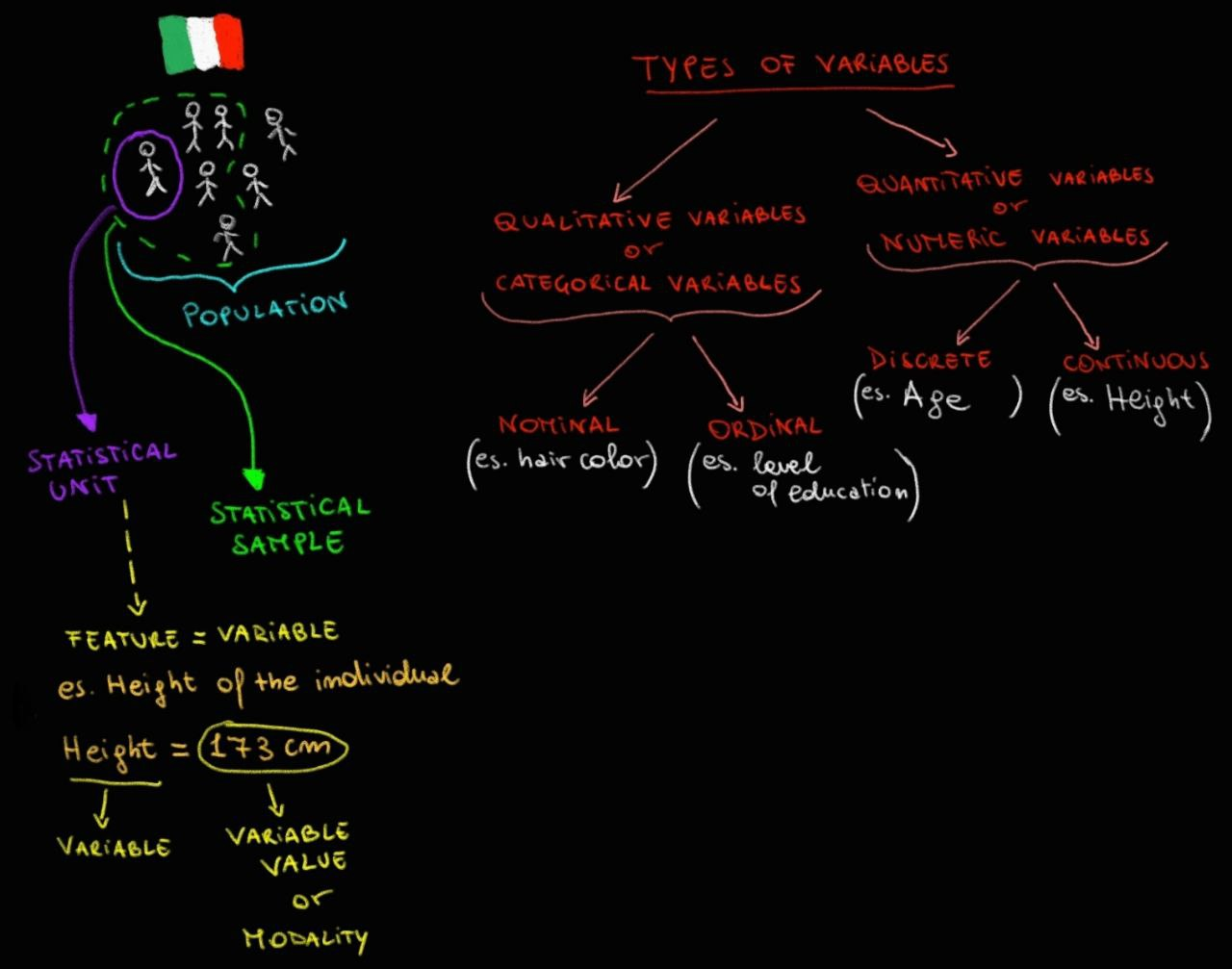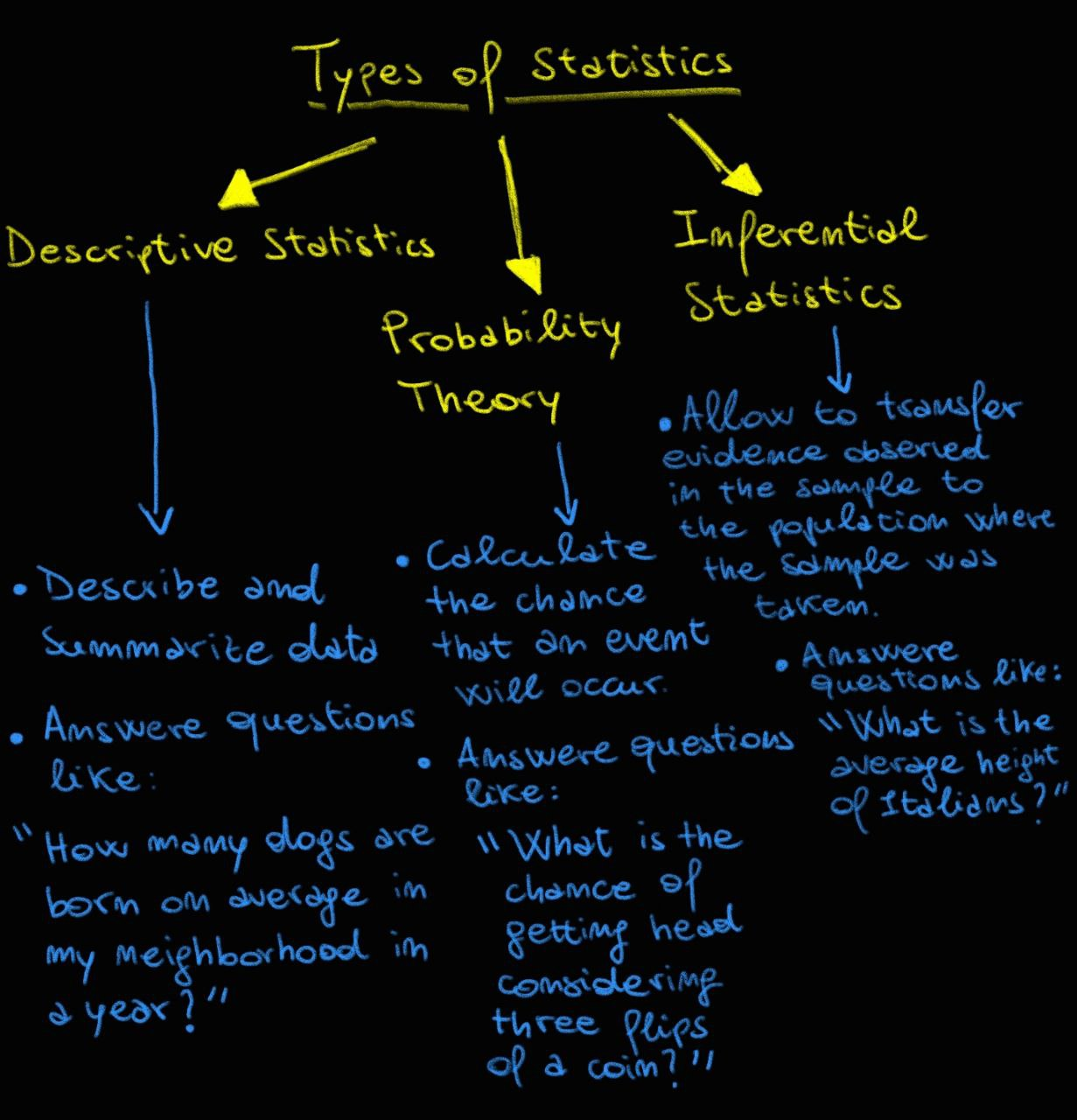Hi,

In the last article, we introduced statistics through an example, illustrating how this discipline can help us objectively answer problems of different nature. Here you can find the link to the article in question and in the image below you can find a summary of the topics covered.Today, I would like to present to you, through this quick article, what I think are the three main branches of statistics, namely descriptive statistics, probability theory and inferential statistics. Each of these branches allows us to answer different types of questions.

Descriptive statistics allows us to answer questions like "What is the average number of children named Mario born in the hospital of Turin between 2020 and 2023?". In fact, descriptive statistics allows us to "describe" the data available, trying to extract summary and general information about them, using tools such as graphs, tables, calculation of absolute and relative frequencies, position indices (e.g. mean, median, mode, quantiles, etc...) and variability indices (e.g. range, interquartile range, variance, standard deviation, Gini's heterogeneity index and coefficient of variation).

Probability theory allows us to calculate the possibility that an event will occur. The possibility is a variable that has values between 0 and 1, or multiplied by 100 to express them in percentages between 0% and 100%. There are different ways to calculate the probability of an event, including classical probability, frequentist probability, and subjective probability. An example of a question that can be answered with probability theory is "What is the probability of getting three consecutive sixes by rolling a dice 20 times?".

Finally, inferential statistics allows us to "infer" or deduce the values of a variable related to a population of statistical units, analyzing a sample of these units. It is therefore possible to transfer the evidence obtained from the observation and analysis of the sample to the population. Inferential statistics allows us to answer questions such as "How is the emergency department of the Milan hospital evaluated by citizens, considering values from 1 to 5, where 1 represents the minimum satisfaction value and 5 the maximum value?".

Well, let's stop here for today. We have discussed the three main branches of statistics and how they can help us answer questions of different nature. Below you can find a summary chart.I remind you that you are free to add considerations, corrections, and improvements in the comments. This would help me and, in general, the community to get the most out of this informative project. I repeat that I write to share what I learn over time and I would be happy to be corrected if necessary, because I would be the first to learn from my mistakes.

Best regards. See you soon.

Omar Almolla